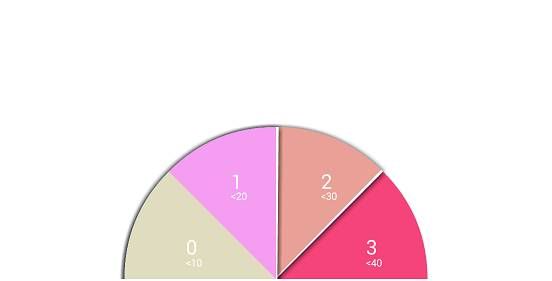# Understanding Sweep angle in drawArc method of android###### Praveena Kumara D

29 Jul 2014It’s always confusing to use a drawArc method in Android. The parameter’s Start Angle and Sweep Angle are quite confusing to understand. This post tries to explain the drawArc function and tries to clear any doubts regarding Start Angle and Sweep Angle parameters.

## How to draw an android draw arc?

You will use Canvas object to invoke a drawArc function which takes four parameters.

public void drawArc (RectF oval, float startAngle, float sweepAngle, boolean useCenter, Paint paint)

Paint attribute decides the style of the Arc (Fill or Stroke etc). Oval parameter decides the shape and size of the arc. Start angle (in degrees) where the arc begins, Sweep Angle measures how long the arc will be drawn and is always measured in clockwise.

## Below 3 points/rules below helps us interpret startAngle and sweepAngle for drawArc method

1. Arc will be drawn in a clockwise direction

2. Below the diagram, indicates the way drawArc assumes degrees over the layout.3. The direction of drawing the arc can be reversed by providing negative angles.

Now it’s time for some examples to better understand how to draw an android arc between two points with respect to Start Angle and End Angle.

Case 1:

startAngle     =    0 degrees

sweep angle  =    90 degreesIn the above diagram, the shaded portion is the rendered arc portion. As you can see, It starts from 0 degrees as depicted in the coordinate system, and it sweeps 90 degrees in a clockwise direction.

Case 2:

startAngle     =    90 degrees

sweep angle  =         180 degrees## Now let’s take a look at the Sample code :

The below code tells you how we are able to draw four arcs starting from a Big arc from 180 start Angle to 180 Sweep Angle. Then the code draws four arcs, each starting at 45 degrees below the previous start angle.canvas.drawArc(rect, 180, 180, false, mSectionPaint4); // draws section 3

canvas.drawArc(rect, 135 180 – section Gap, false,mSectionPaint3); // draws section 2

canvas.drawArc(rect, 90, 180, false, mSectionPaint2);     // draws section1

canvas.drawArc(rect, 45, 180, false, mSectionPaint1);  // draws section 0

You can check out a Custom view called ArcProgressBar from our repository here: https://bitbucket.org/pkumarad/arcprogress